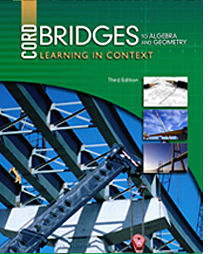# Bridges to Algebra and Geometry - 2nd Edition

## Chapter 10: Introduction to Geometry

### 10.1 Basic Geometric Figures

http://www.mcwdn.org/Geometry/Lines.html
NEW This page defines the basic geometric shapes needed for this course, and a link to a practice quiz (uses Java for scoring).

http://www.mathsisfun.com/geometry/line.html
NEW Some important facts about lines and line segments.

### 10.2 Angles

http://www.mathsisfun.com/angles.html
NEW This page defines the different kinds of angles and has several practice problems.

http://www.mathplanet.com/education/geometry/points,-lines,-planes-and-angles/measure-and-classify-an-angle
NEW This site shows how to measure and classify angles.

http://www.explorelearning.com/index.cfm?method=cResource.dspResourcesForCourse&CourseID=222
This site has examples and problems for students to solve that involve lines and angles.

### 10.3 Angle Relationships

http://www.mathsisfun.com/geometry/parallel-lines.html
NEW This page and others linked here define and give practice identifying the many angles formed by lines crossing parallel lines.

http://www.regentsprep.org/Regents/math/geometry/GP8/indexGP8.htm
NEW This site includes a couple pages discussing and practicing the properties of angles associated with parallel lines.

http://www.math10.com/en/geometry/angles/angles.html
NEW This page includes practice naming and identifying angles formed by a line intersecting parallel lines.

### 10.4 Triangles

http://www.math.com/school/subject3/lessons/S3U2L2GL.html
This site defines triangles and explains how to classify them.

http://www.mathsisfun.com/triangle.html
NEW This site provides some interactive learning and practice problems involving triangles.

### 10.5 Polygons

http://www.explorelearning.com/index.cfm?method=cResource.dspResourcesForCourse&CourseID=223
This site has examples and problems for students to solve that involve quadrilaterals and other polygons.

http://www.math.com/school/subject3/lessons/S3U2L1GL.html
This site defines polygons and its components and has a table of the properties of polygons with up to 10 sides.

### 10.6 Congruence

http://www.mathsisfun.com/geometry/congruent.html
NEW This site prepares you for the lessons to come where the definition of congruence is important. It includes some practice problems.

### 10.7 Translations

http://www.explorelearning.com/index.cfm?method=cResource.dspResourcesForCourse&CourseID=251
This site has examples and problems for students to solve that involve transformations.

http://www.regentsprep.org/Regents/math/geometry/GT2/Ltrans.htm
NEW This site presents an intuitive understanding of translations. More advanced content is available, if desired.

http://www.mathsisfun.com/geometry/translation.html
NEW This page provides some interactive learning and practice problems involving translations.

### 10.8 Reflections

http://www.explorelearning.com/index.cfm?method=cResource.dspResourcesForCourse&CourseID=251
This site has examples and problems for students to solve that involve transformations.

http://www.regentsprep.org/Regents/math/geometry/GT1/Lreflect.htm
NEW This site presents an intuitive understanding of reflections. More advanced content is available, if desired.

http://www.mathsisfun.com/geometry/reflection.html
NEW This page provides some interactive learning and practice problems involving reflections.

### 10.9 Rotations

Transformations
This site has examples and problems for students to solve that involve transformations.

http://www.regentsprep.org/Regents/math/geometry/GT4/Lrotate.htm
NEW This site presents an intuitive understanding of rotations. More advanced content is available, if desired.

http://www.mathsisfun.com/geometry/rotation.html
NEW This page provides some interactive learning and practice problems involving rotations.